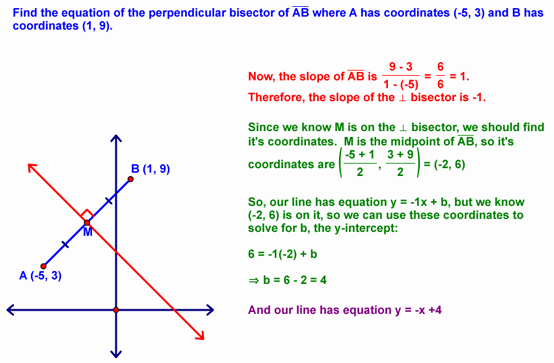you are here > Class Notes - Chapter 13 - Lesson 13-Printable version

Equations of Lines - Lesson 13-2

We next recalled how to graph horizontal and vertical lines:Which is summarized as follows:We also learned today how to determine the equation of a line in y = mx + b format. This is a two step process:

1. Determine the slope (m). This is often accomplished by finding the slope of a line that we know to be parallel or perpendicular to the line we're trying to define. It can also be done by using the slope formula if we know the coordinates of two points on the line.
2. Determine the y-intercept. This is accomplished by plugging the coordinates of a point we know is on the line into the equation y = mx + b (remember that we already know m) and solving for b.

So, for example...we might do the following:Other LinksClass NotesLesson 13-1Lesson 13-2Lesson 13-3Lesson 13-4Lesson 13-6Quiz TopicsIf you have questions, email me at baroodyj@doversherborn.org